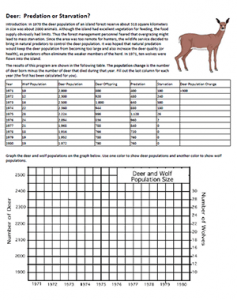# Deer: Predation or Starvation?This activity asks students to calculate the population change (births – deaths) and then graph the number of deer and the number of wolves.   An introductory paragraph describes a situation where deer were overpopulated and were in danger of starvation due to lack of food.  To solve this problem, predators were introduced.  The graph will show a decline in the deer population after the predators were introduced but also due to starvation.

Analysis questions ask to students to summarize the relationship between wolves and deer and to make judgments about whether wildlife management should include the reintroduction of predators.

Zero population growth (ZPG) is also defined and students must determine which year was closest to ZPG and then discuss their opinion about whether it as a good idea to introduce the wolves.

1.2.